Rotating Clock Divider [RCD] Rotating Clock Divider < Modules < Eurorack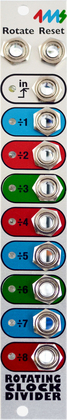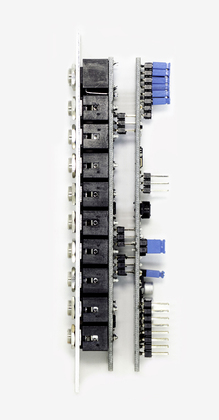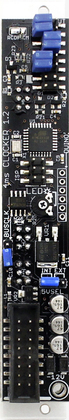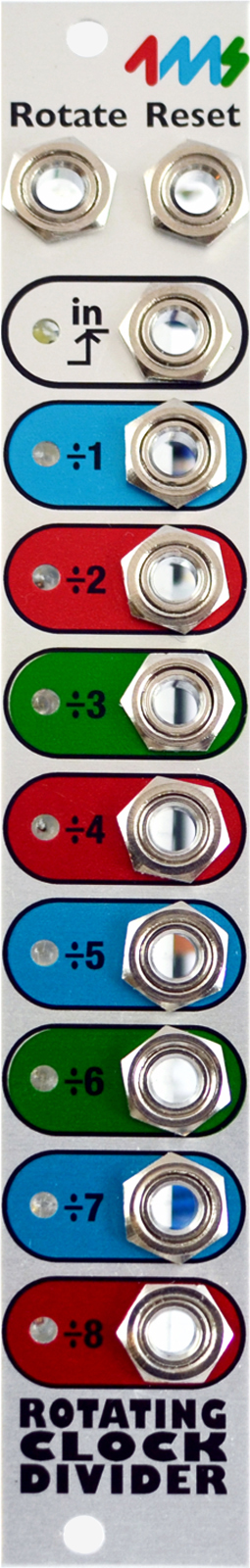The Rotating Clock Divider (RCD) produces eight divided clock tempos from a single input clock. The divisions range from /1 to /64, including all odd and non-standard divisions such as /3 and /17 and /62. A Rotate CV input re-assigns the division number of each jack, allowing for creative mix-ups and experimentation. A Reset input jack helps to sync/start with an external clock.

\$179.00

is: display:block; Basic Features:
• Divide-by-1 to Divide-by-64, on 8 output jacks
• CV Rotate jack to shift divide-by amount on each jack
• CV Reset jack to reset/re-sync all jacks
• Optional break-out panels to expand functionality (Auto-reset, selectable Divide-by range, Gate/Trigger, Up/Down-counting)
Jacks:
• Clock Input (5V to 15V clock, rising edge triggered), max 3kHz
• CV Rotate (0V to +5V input)
• CV Reset (5V to 15V trigger)
• Divided Clock Outputs (8 jacks):
• Divide-by-(1+R)
• Divide-by-(2+R)
• Divide-by-(3+R)
• Divide-by-(4+R)
• Divide-by-(5+R)
• Divide-by-(6+R)
• Divide-by-(7+R)
• Divide-by-(8+R)
...where R is the CV Rotation.
• Selectable Gate/Trigger mode:
• Selectable Divide range up to Divide-by-64:
Two jumper pins on the back can be pre-set with jumpers plugs, or used with (forthcoming) breakout panel.
• Selectable Auto-reset:
Auto-reset will reset the divide counter every 16/24/32 clocks, which keeps the beats more "dance-able" even when using the "weird" clock divisions (3/5/6/7/9/10/11/etc..) Two jumper pins on the back can be pre-set with jumpers plugs, or used with (forthcoming) breakout panel.
Electrical and Mechanical Specifications:

• Module size:
• 4 HP Eurorack format module
• 1.39" (35mm) deep
• Power consumption:
• 5VSEL Jumper set to INT:
• 33mA maximum power draw from +12V rail
• 0mA max on the +5V rail
• 4mA maximum power draw on the -12V rail
• 5VSEL Jumper set to EXT:
• 19mA maximum power draw on the +12V rail
• 14mA max on the +5V rail
• 4mA maximum power draw on the -12V rail
Included with the RCD:
• 16-to-16 pin power cable
• 2 M3 Knurlie screws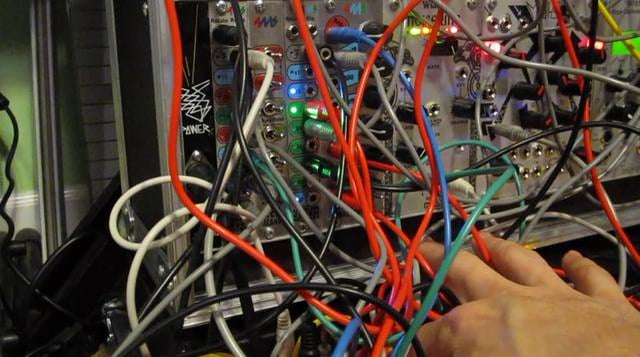Richard Devine: Tripping Beats with RCD SCM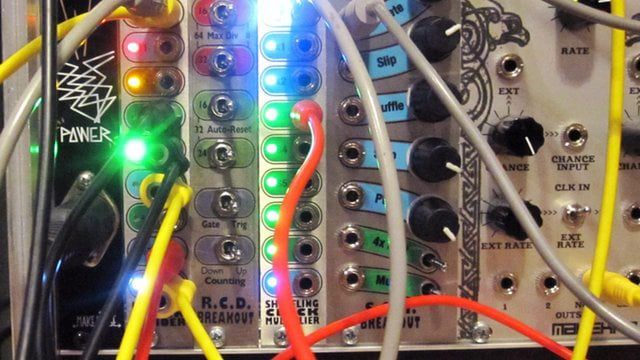Richard Devine: Mutant Mech Dub with RCD and SCM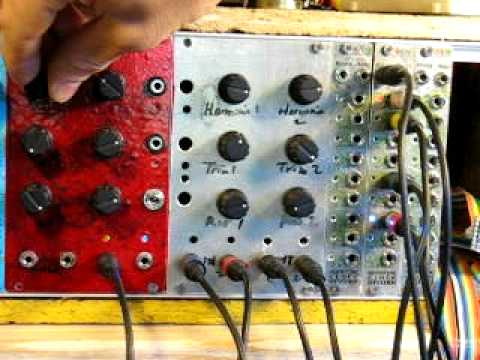Rotating Clock Divider - CV RotateBanana Frac Rotating Clock Divider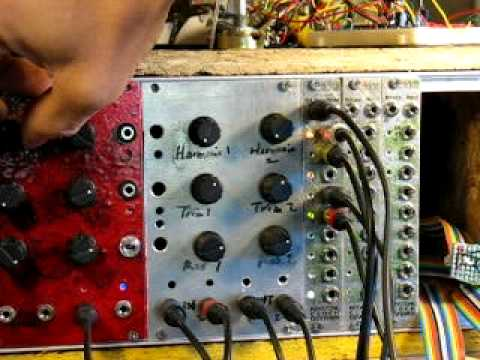Rotating Clock Divider - Autoreset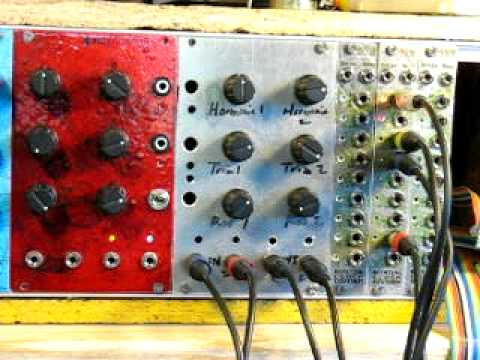Rotating Clock Divider - Reset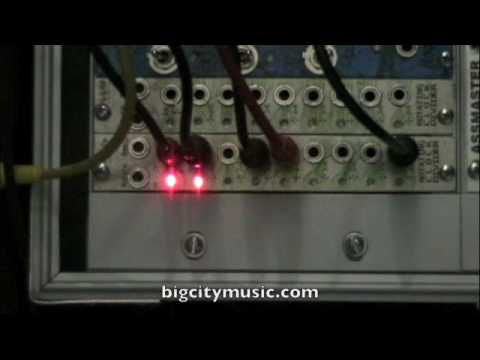BCM demo Rotating Clock Divider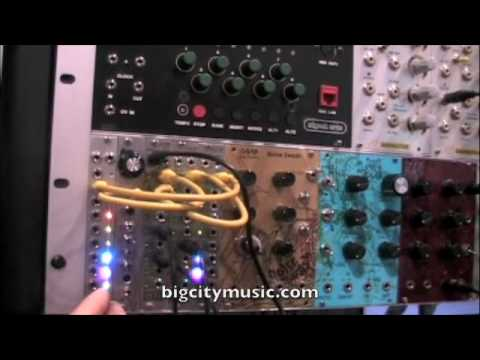Rotating Clock Divider at NAMM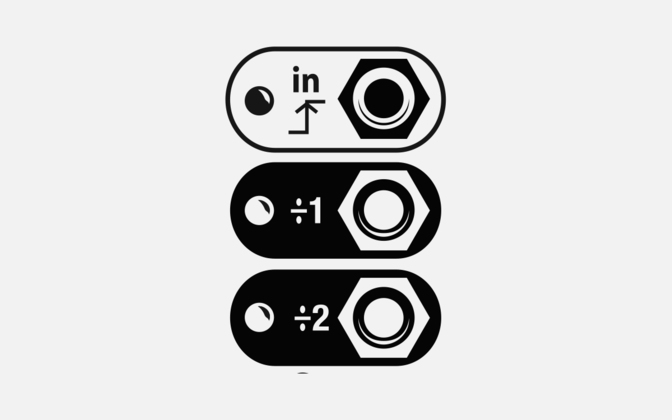−